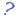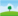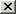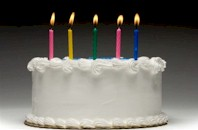# Les numéraux ordinaux: 1-10 (The ordinal numbers)

## Complétez les phrases par les adjectifs numéraux ordinaux corrects. (Fill in the blanks with the correct ordinal numbers.) Pour voir la liste des nombres ordinaux cliquez ici. (Click here to get information about the ordinal numbers.) Cliquez surpour avoir de l'aide. (Click on the question mark to get help.)

1. Paris compte 2.240.621 habitants, Marseille 852.516 et Lyon, avec ses 496.343 habitants est laplus grande ville de France.
2. Le mois d'août est lemois de l’année et le mois de septembre le .3. LaGuerre mondiale a débuté en 1914 et laa commencé en 1939.
4. Aujourd’hui c’est leanniversaire de Simon.
5. Le lundi est le premier jour de la semaine, le jeudi est leet le samedi le .6. En général, les bébés naissent pendant le neuvième ou lemois de la grossesse.
7. On appelle le cinéma leart.

3.
Cf. Les numéraux ordinaux.

The third.
Consult the following information: The ordinal numbers8.
Cf. Les numéraux ordinaux.

The eighth.
Consult the following information: The ordinal numbers9.
Cf. Les numéraux ordinaux.

The ninth.
Consult the following information: The ordinal numbers1.
Cf. Les numéraux ordinaux.

The first.
Consult the following information: The ordinal numbers2.
Cf. Les numéraux ordinaux.

The second.
Consult the following information: The ordinal numbers5.
Cf. Les numéraux ordinaux.

The fifth.
Consult the following information: The ordinal numbers4.
Cf. Les numéraux ordinaux.

The fourth.
Consult the following information: The ordinal numbers6.
Cf. Les numéraux ordinaux.

The sixth.
Consult the following information: The ordinal numbers10.
Cf. Les numéraux ordinaux.

The tenth.
Consult the following information: The ordinal numbers7.
Cf. Les numéraux ordinaux.

The seventh.
Consult the following information: The ordinal numbers